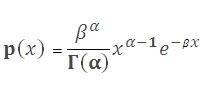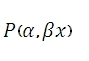# SQL Server GAMMA.DIST function

Updated: 9 August 2010

Use GAMMADIST to calculate the gamma distribution. The formula for the probability density function of the gamma distribution is:And the cumulative distribution function is the incomplete gamma functionSyntax
<@X, float,>
,<@Alpha, float,>
,<@BETA, float,>
,<@Cumulative, bit,>)
Arguments
@X
is the value at which you want to evaluate the distribution. @X is an expression of type float or of a type that can be implicitly converted to float
@Alpha
is the alpha parameter to the distribution. @Alpha is an expression of type float or of a type that can be implicitly converted to float
@BETA
is the beta parameter to the distribution. @BETA is an expression of type float or of a type that can be implicitly converted to float
@Cumulative
is a logical value that determines if the probability density function (False, 0) or the cumulative distribution function (True, 1) is being calculated.
Return Types
float
Remarks
·         If @X < 0, GAMMADIST will return an error
·         If @Alpha ≤ 0 or @BETA ≤ 0, GAMMADIST will return an error.
Examples

select

This produces the following result
----------------------
0.032639130418294

(1 row(s) affected)

select

This produces the following result
----------------------
0.0680940037098249

(1 row(s) affected)

select
wct.GAMMAP(9,10.00001131/2)

This produces the following result
----------------------
0.0680940037098249

(1 row(s) affected)Copyright 2008-2023 Westclintech LLC         Privacy Policy        Terms of Service